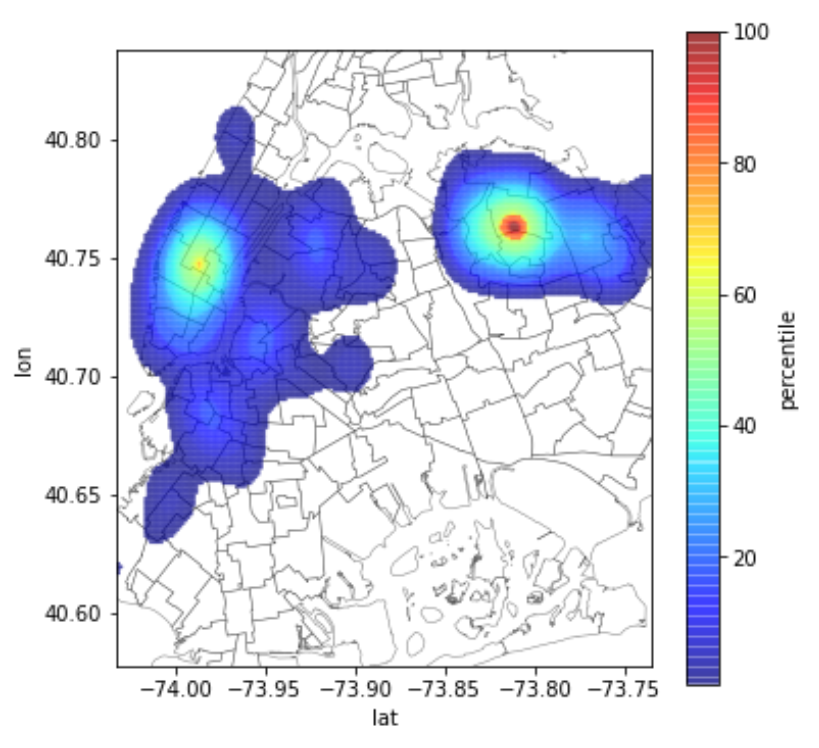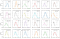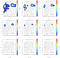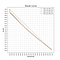# Estimating the Geographic Area of a Real Estate Agent

[Special thanks to Compass’s NYC_AI team and to Hezhi Wang!]

At Compass, many of our current and envisioned products and services require knowledge of whether a location or geographic area is relevant to a particular agent, and conversely, who are appropriate candidate agents for a home or area.

For example, we have millions of prospective likely-to-sell homes that are not already associated with an agent’s CRM contacts. To which agent or agents should we recommend each of these properties? Knowing that the agent does significant work in the area around the home is an important factor for this decision.

A similar example is when we receive “I’m Interested” leads from visitors to Compass.com. To which agents should we route a particular lead? Routing algorithms again take into account various factors to ensure fair allocation, and one of those factors is whether the agent does significant work in that area.

Another example is when an agent’s seller client also is going to buy a home (not unusual), but they’re going to buy in an area where the agent does not work — for example, in a different city or state. The Compass platform should help locate the right agent for their client’s buying transaction. Knowing which agents have the strongest presence in the target location is one key factor.

Below, we describe how we approach the problem, train our models, evaluate them, and provide some hints for efficient implementation. Let’s call this “Area of Agent” modeling.

Simplistic Area of Agent models, unfortunately, result in undesirable behavior. For example, in the past, we have used “an agent has had at least k transactions in this zipcode.” Agents have criticized the results of products based on such models, pointing out that for various reasons they conduct small amounts of business in areas where they do not generally work.

More importantly, using arbitrary predefined boundaries, like zipcode, produces undesirable effects. In New York City, zipcodes 10012 and 10013 abut each other and have relatively similar properties and clientele. However, we see many agents who are “above threshold” in one of the two and not the other. It would make more sense to avoid the arbitrary zipcode boundaries already and instead to see how concentrated the agents’ work is in the general area. For large zipcodes (or other arbitrary boundaries), we can also see the opposite effect: within the zip there are areas where an agent works and other areas where they do not.Figure 1. The Area of Agent for an NYC Agent who works primarily in Queens and Midtown Manhattan, but also does business in lower Manhattan and western Brooklyn

Instead, we would prefer a continuous geographic representation of the strength of an agent’s business, such as is shown in Figure 1 for an agent working in New York City.

# The Goal

The goal of our Area of Agent modeling is to measure the “market” where each agent is active. We will focus on the geographical area in this post, but the approach is easily generalizable to include other factors, such as price range, types of houses, etc.

To model the area of an agent, we take various inputs, such as:

• past transactions that the agent worked on
• the geographic locations of their clients

These inputs become a set of points represented by their latitude and longitude. For example, let’s assume that this is the map of the past transactions for the agent in NYC.

# More Technically...

Our goal is to decide whether a location is within or outside the agent’s area of business activity. Technically, we want to get back a normalized score, with 0 being “not within the area” and 1 being “the best possible point.”

A related task is, given a location or area, to return the most appropriate agents.

(As a note, modeling Area of Agent has been one of our most frequently used data science interview questions. It is simple enough so that any scientist can tackle it within the timeframe of an interview, but also “unusual” enough that people without sufficient data science/machine learning depth fail spectacularly. An interview question that keeps on giving.)

# Would a geographic histogram work?

A basic approach for estimating the area for an agent would be to construct a two-dimensional histogram, based on some predefined “buckets”, and count the number of past transactions that fall within each bucket. For example, we could count the number of sales within a county, city, zip code, or even within a census block.

Unfortunately, using histograms as Area of Agent models present problems.

First, choosing the granularity of the histogram is problematic: too coarse, and we lose the ability to inform decisions, too fine, and we end up with buckets with only one or zero observations in them. Furthermore, the right granularity depends on the area. Using counties may be ok in some locations but too broad in others. Zipcodes might be ok within NYC, too fine-grained for Atlanta and Seattle, and too coarse for the Hamptons or Lake Tahoe. Census blocks can be ok for some agents that focus on small areas — such as a few large buildings in NYC — but are too fine-grained for agents that cover much broader areas.

Second, and most importantly, using a simple histogram to draw inferences about buckets that have no prior observations would typically result in a prediction of zero. Thus, at the very least a histogram would have to be augmented with some sort of statistical “smoothing,” but deciding how to smooth the values across neighboring buckets is tricky.

For example, below, we can see a two-dimensional histogram example from Wikipedia, with two different estimations for precisely the same dataset of x-y values. The only difference is the placement of the boundaries for the buckets. Move the grid a bit without changing anything else, and the resulting area estimation changes drastically.

# The Solution: Multivariate Kernel Density Estimation

Instead of relying on histograms, we decided to use multivariate kernel density estimation (KDE). For an introduction to kernel density estimation, the visualization by Matthew Conlen is excellent. For more details on multidimensional density estimation, read the Wikipedia article and the sklearn tutorial.

The basic idea behind KDE is to assign a “kernel” to each of the data points. A kernel is a function that (roughly) helps statistical and machine learning methods to determine similarity. One of the most common kernel functions is the Gaussian kernel, and, in the 2-dimensional case, it might look like this:

Here, if we started with a point in the middle, the kernel function would say that other points are less and less similar as they land on larger concentric circles. In other words, if we interpret this geographically, it would mean that points that are further away are less similar. A key aspect of this kernel function is that it takes a point (in the center) and spreads it out. The strength decreases radially outward, with the contour lines showing equal strength.

That Gaussian was circular. If we configure the kernel with unequal variance in the x- and y-axis, it might look like this:

That would say that similarity is not simply constant with respect to “birds fly” distance. (Compare this to the contours of a topological map, where similarity is represented by equal altitude and may have a very irregular shape.)

Ok, so that’s just one instance of a kernel function. A KDE model combines many kernels — one for each input data point. In our example, that could be one for each past transaction or client address. The collection of kernels would look like this. The idea is that each data point (e.g., each past transaction) spreads its “influence” to the nearby area, following the shape of the kernel:

Finally, to produce an Area of Agent model, we sum up the kernel densities of the individual points. The result is the kernel density estimation of all the points together:

The colors show how strongly a particular geographic spot associates with the agent, with red being the strongest and white the weakest. Note that the red area is red because (a) there are a lot of transactions in that area, and (b) the kernels from those points fill in the gaps and reinforce each other when they are summed.

At this point, we have assigned a density (strength) value to all parts of the space.

The following questions remain:

• How do we select the kernel function to use, and how do we choose the parameter values for the kernel functions? The parameter values would determine things like how much to spread out each point.
• How do we go from the kernel density values into a final score, deciding whether any particular geographic location is “inside” or “outside” the agent’s area, and if inside, how strongly associated with the agent?

# What kernel function to use?

While we certainly could experiment with fancy functions, in our case, we stick with a simple 2d Gaussian function, without covariance and with equal variance across the x and y-axis. In other words, a kernel function like this:

The idea behind this simple choice is twofold: (i) it captures the idea that geographic proximity is the primary building block for modeling Area of Agent, and (ii) we will rely on the summing of multiple kernels to shape the areas for particular agents.

There is still a crucial parameter to choose: The “variance” of the Gaussian — essentially, how spread out the Gaussian should be. This parameter is called the “bandwidth” of the kernel.

If we pick the bandwidth to be very large, we will have a very “spread out” estimate. On the other hand, if we choose the value to be too small, then our area estimate will comprise only our observed data points.

Here are some examples of different bandwidth settings for the NYC transactions that we showed above. Remember, the points looked like this:

First, an estimate with a bandwidth that seems too large:

That says that the agent works in (almost) all of Manhattan, Brooklyn, and Queens.

Then, one with a bandwidth that is too small:

That suggests that the agent only works right in the specific locations where they previously transacted.

And with the Goldilocks (just right) bandwidth setting (we will explain):

# How to get the bandwidth parameter “just right”?

Recall that the bandwidth value spreads out the individual transaction activity, both filling in the gaps between individual spots and also extending the transactions to neighboring areas.

Deciding the proper bandwidth value is a critical part of the estimation. There are many techniques for estimating the right kernel bandwidth. So, let’s not lose sight of our business goal. We want to make sure that our estimation does the best job we can of representing where our agent works. We could proxy for this with the following simple test: if we naturally watched where the agent worked next, would those locations fall within our estimated area?

We can simulate this test in the usual machine learning way: separate our data into some data used to build the model (the training data) and some data to evaluate the model (the test data).

Then, to estimate the optimal value for the bandwidth parameter for an agent, we use a maximum likelihood approach: We split our training data randomly into two subsets, “training” and “validation”:

• We “train” our model using various bandwidth values s on the training data points.
• Using the KDE estimated with bandwidth s, we estimate the density for all points in the validation set.
• We pick the value s that maximizes the likelihood of the data points in the validation set.

To have a robust estimate for the “optimal” value of s, we can repeat the process multiple times. Once we have estimated the right bandwidth value for an agent, we store it for future use. Importantly, note that we have a different bandwidth value for each agent, as individual agents tend to have different levels of geographic focus.

# Bandwidth values as a function of geographic area

The bandwidth value for each agent tends to capture how broad the geographic area the agent covers is, controlling for the number of transactions. Small values mean that the agent is very focused on particular geographic locations and vice versa. However, what is a “particular geographic location” varies widely across cities. The plot below shows how the bandwidth values of agents vary by broad geographic area:The x-axis is the log(bandwidth), and the y-axis shows the density of agents with that bandwidth value. Distributions centered to the left have more geographically focused agents, and vice versa for those centered to the right in their plots. The Hamptons, Lake Tahoe, NYC, and Boston have very geographically focused agents. Seattle, Atlanta, Los Angeles, and the Bay Area (sf) have the most geographically spread out agents.

# From densities to normalized scores

The process above generates a two-dimensional density distribution over latitude/longitude (lat, lon) values.

This isn’t the greatest for our applications, because density values of continuous probability distributions tend to be hard to interpret: Individual density values can have values above one and do not have a direct probabilistic interpretation. And even the statistically minded have difficulty explaining what they mean to non-experts.

To allow the density scores to be more interpretable, we normalize the density values. We apply the following normalization process:

• We take all the data points in the training data and estimate their density values using the model
• We estimate the empirical distribution of density values for all the instances in the training data
• We apply percentile normalization to the density values

So, now, if we query the model with a new value (lat, lon), we get back a value ranging from 0 to 1. The interpretation is straightforward: A value of 0 means that all the training data points have density values higher than the query data point. A value of 0.1 means that 10% of the training data points have density values lower than the query data point, and so on.

We can start to see how these normalized scores will be useful for our various applications. For example, we can use the percentile to define the “top-x%-area-of-agent”. The x% means that we expect that x% of the agent’s transactions will fall within that area. This gives an adjustable area for each agent, that is not based on arbitrary location boundaries. Below, you can see a plot of how our example NYC agent’s estimated area changes as we change the threshold from top-100% (broadest) to top-20% (more focused). What would be the right area to use would depend of course on the application.The shaded area in the upper right corner plot captures all areas: that is the “top-100% area of agent”. Then we show the area with a normalized score “top-90%”, “top-80%”, and up to “top-20%” (in the lower right corner).

# Evaluating Area of Agent models: Predictive value and calibration

One attractive aspect of the final definition of the Area of Agent scores is that they are straightforward to evaluate. The models cast the Area of Agent for any bandwidth setting as the percentage of the agent’s transactions that fall within the area. Therefore, we can pose a predictive task to evaluate. Given an x%-area-of-agent estimate, what if we looked at that agent’s next transaction? Does it have an x% chance of falling in that area?

To test that, we held out each agent’s latest transactions as a test instance. After the model was built, we revealed the test instances and scored them (using the normalized model for the corresponding agent for each).

We then measured what fraction of the test transactions had a score above any given threshold. In principle, when we mark transactions with a score of “top-90%” as “within area”, then we should have a “recall” of 90% (i.e., the models should mark 90% of the transaction as “in area”).

Similarly, when we only mark transactions with “top-20%” and above as “within area,” we should have a recall equal to 20%.

Here is the recall/calibration curve that we observed:The recall calibration curve shows that the normalized score is well-calibrated. However, around 8% of the agent transactions tend to be in a “new area” that does not match the prior transactions. We also experimented with various splits between training/validation, to ensure that our results are consistent for different amounts of training data, and we did not notice significant differences.

You’ll see from the curve that the models are fairly well-calibrated. There is a smooth and straight relationship between where the model predicts that the next transactions will be, and where the transactions are. This means that as we spread out in the agent’s estimated area, essentially going to cooler colors in the heatmap, we indeed see a graceful reduction in the likelihood that the next transaction will be in that area. (It is not represented in the figure, but generally, the size of the areas see accelerating growth with decreasing threshold, so we are needing increasingly larger areas to capture a constant number of additional transactions.).

The dotted line in the figure above shows what perfect calibration would look like. We see that our models are not perfectly calibrated, and we can see exactly why. The almost vertical 10% segment at the left end of the recall curve shows that 10% of the agents’ transactions come from locations that essentially have not been observed before — new areas that do not fall into even “smoothed” areas from prior transactions.

You might notice that we can improve our recall values by playing a simple trick: increase the bandwidth, and therefore, increase the area of the agent. We do not have clear negative examples, so there is no direct way to measure how just broadening the area hurts the model — for example, in other machine learning contexts we might see lower precision.

In such scenarios, instead of precision, we use the concept of model “efficiency.” Efficiency is how compact is the area that we predict. So, for example, if we predict that the entire globe is the “area of the agent,” we will have 100% recall — every possible future listing will be in the area of the agent! (However, notice that getting good calibration across the different quantiles is not so easy. Presuming that we want to represent the AoA as a small set of contiguous regions, it is not so easy to say how you would get 50% recall … or 80% or 20%.)

More than just being able to achieve good calibration, though, the goal of efficiency is to be able to get a particular level of recall with as tight an area as possible. If you look at the “20% recall” plot above, it finds a particular, small area in Queens where this agent does 20% of their business.

More specifically, we measure efficiency as follows:

• Define the baseline area of the agent as the “bounding box” that contains all the past transactions.
• Efficiency is the fraction of the bounding box that we classify as “x% area of the agent.”
• Define recall as the percent of transactions classified to be within the “x% area of agent” for various values of x.

The resulting curves (on a per-agent basis) look like this.

The “area above the curve” — assuming the vertical range of the curve is scaled to 1.0— is a normalized measure of the performance for the model on a per-agent basis. A score close to 1 means that we can predict very well the areas of future transactions while covering a tiny part of the map. A value of 0 results from just saying the whole bounding box is always the area. And if you flip a (weighted coin) to determine if any point is in or out of the area, you would get the diagonal line (that’s more of a technical point — it’s not something that one would consider an “area”).

# Use Cases

So, where can we use these geographic area profiles?

While the initial use cases are to provide our agents with the most appropriate listings and leads and referral clients, the same model functionality is helpful for a variety of other use cases:

• Does a (prospective) listing fall within the Compass area?
• How similar are these agents based on their geographic area of activity?
• How would a prospective new addition to the Compass agent corps add to the company’s geographic footprint?
• When we suggest an address for an existing CRM contact, does the address make sense for this agent?

# Future Steps

The described approach is a new way to apply fairly straightforward kernel density estimation. There are various extensions that we can consider:

• Extend the notion of “area of agent” to include the price ranges where the agent operates. This is interesting because at a simple level, price is one-dimensional, so the kernel estimation is easier. On the other hand, there may be a sophisticated interaction between location and price. An agent may not be willing to go “way over there” for a listing in their normal price range, but if it would extend their experience on the upside, maybe so...
• Extend the querying capability score entire areas and not only lat/lon point queries. This could involve taking the area as input and then “integrating” the density function over the area. This could allow selecting the most appropriate agents for, say, a buyer lead who specifies that they would like to buy in a particular area.
• Allow for faster estimation of the area of an agent, using only a small number of transactions. In a future blog post, we will show how we can use autoencoders for this task.

## Compass True North

Compass Engineering & Product Blog — An inside glimpse at…

## Compass True North

Compass Engineering & Product Blog — An inside glimpse at our technology and tools, brought to you by the engineers of the game-changing real estate platform, Compass. Hiring at https://www.compass.com/careers/

Written by

## AI@Compass

The AI@Compass Team is building the AI to support the first end-to-end tech platform for real estate. This is our story.## Compass True North

Compass Engineering & Product Blog — An inside glimpse at our technology and tools, brought to you by the engineers of the game-changing real estate platform, Compass. Hiring at https://www.compass.com/careers/